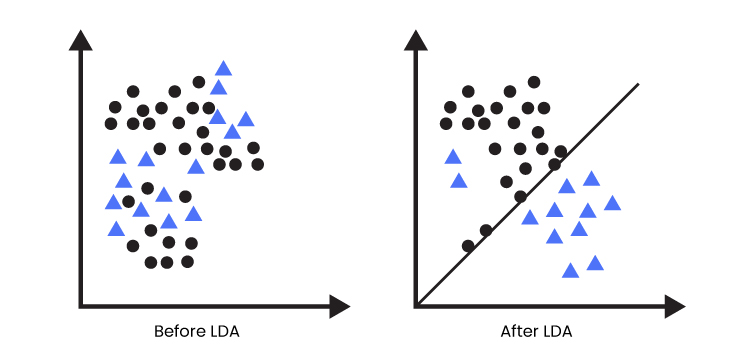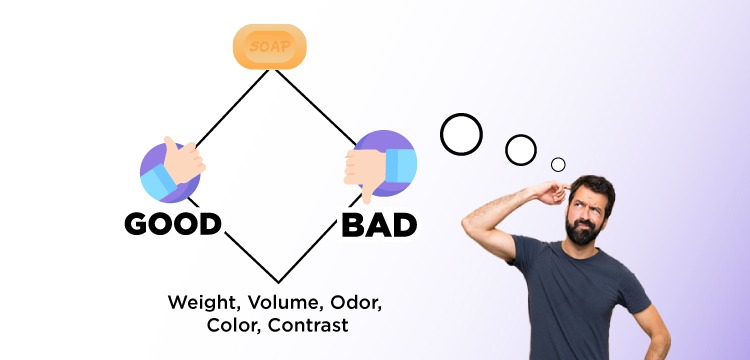• Category
• >Machine Learning

# Introduction to Linear Discriminant Analysis in Supervised Learning

• Neelam Tyagi
• Nov 28, 2019
• Updated on: Mar 04, 2021With the advancement in technology and trends in connected-devices could consider huge data into account, their storage and privacy is a big issue to concern.

Data hackers make algorithms to steal any such confidential information from a massive amount of data. So, data must be handled precisely which is also a time-consuming task.

Also, we have seen, not all the data is required for inferences, reduction in data-dimensions can also help to govern datasets that could indirectly aid in the security and privacy of data.

In the core aspects of this blog, we will dwell on data dimensionality reduction techniques, it will cover the concept of Linear Discriminant Analysis(LDA), the difference of LDA and PCA and related applications.

## Introduction to LDA

In 1936, Ronald A.Fisher formulated Linear Discriminant first time and showed some practical uses as a classifier, it was described for a 2-class problem, and later generalized as ‘Multi-class Linear Discriminant Analysis’ or ‘Multiple Discriminant Analysis’ by C.R.Rao in the year 1948.

Linear Discriminant Analysis is the most commonly used dimensionality reduction technique in supervised learning. Basically, it is a preprocessing step for pattern classification and machine learning applications.

It projects the dataset into moderate dimensional-space with a genuine class of separable features that minimize overfitting and computational costs.

With the aim to classify objects into one of two or more groups based on some set of parameters that describes objects, LDA has come up with specific functions and applications, we will learn about that in detail in the coming sections.

(Suggested blog: Machine Learning Algorithms)

Under Linear Discriminant Analysis, we are basically looking for

1. Which set of parameters can best describe the association of the group for an object?

2. What is the best classification preceptor model that separates those groups?

It is widely used for modeling varieties in groups, i.e. distributing variables into two or more classes, suppose we have two classes and we need to classify them efficiently.Classification of various objects before and after implementing LDA

Classes can have multiple features, using one single feature to classify may yield in some kind of overlapping of variables, so there is a need of increasing the number of features to avoid overlapping that would result in proper classification in return.

(Must Read: Top Machine Learning Tools)

Here is the the video that clearly explains LDA

### Example of LDA

Consider another simple example of dimensionality reduction and feature extraction, you want to check the quality of soap based on the information provided related to a soap including various features such as weight and volume of soap, peoples’ preferential score, odor, color, contrasts, etc.

A small scenario to understand the problem more clearly;

1. Object to be tested -Soap;

2. To check the quality of a product- class category as ‘good’ or ‘bad’( dependent variable, categorical variable, measurement scale as a nominal scale);

3. Features to describe the product- various parameters that describe the soap (independent variable, measurement scale as nominal, ordinal, internal scale);Pictorial view of an object, class category, and features extraction

When the target variable or dependent variable is decided then other related information can be dragged out from existing datasets to check the effectivity of features on the target variables.

And hence, the data dimension gets reduced out and important related-features have stayed in the new dataset.

(Related reading: Clustering methods and application)

### Extensions to LDA:

1. Quadratic Discriminant Analysis (QDA): Each class deploys its own estimate of variance, or the covariance where there are multiple input variables.

2. Flexible Discriminant Analysis (FDA): Where the combinations of non-linear sets of inputs are deployed such as splines.

3. Regularized Discriminant Analysis (RDA): It adds regularization into the estimate of the variance, or covariance that controls the impact of various variables on LDA. (Source)

Moreover, the limitations of logistic regression can make demand for linear discriminant analysis.

### Limitations of Logistic Regression

Logistics regression is a significant linear classification algorithm but also has some limitations that leads to making requirements for an alternate linear classification algorithm.

• Two-Class Problems: Logistic regression is proposed for two-class or binary classification problems that further be expanded for multi-class classification, but is rarely used for this purpose.

• Unstable With Well Separated Classes: Logistic regression is restricted and unstable when the classes are well-separated.

• Unstable With Few Examples: Logistic regression behaves as an unstable method while dealing with few examples from which parameters are estimated.

Linear Discriminant Analysis can handle all the above points and acts as the linear method for multi-class classification problems.

## Working of Linear Discriminant Analysis

### Assumptions

1. Every feature either be variable, dimension, or attribute in the dataset has gaussian distribution, i.e, features have a bell-shaped curve.

2. Each feature holds the same variance, and has varying values around the mean with the same amount on average.

3. Each feature is assumed to be sampled randomly.

4. Lack of multicollinearity in independent features and there is an increment in correlations between independent features and the power of prediction decreases.

While focusing on projecting the features in higher dimension space onto a lower dimensional space, LDA achieve this via three step process;

1. First step: To compute the separate ability amid various classes,i.e, the distance between the mean of different classes, that is also known as between-class variance.1. Second Step: To compute the distance among the mean and sample of each class,that is also known as the within class variance.1. Third step: To create the lower dimensional space that maximizes the between class variance and minimizes the within class variance.

Assuming P as the lower dimensional space projection that is known as Fisher’s criterion.## Application of Linear Discriminant Analysis

There are various techniques used for the classification of data and reduction in dimension, among which Principal Component Analysis(PCA) and Linear Discriminant Analysis(LDA) are commonly used techniques.

The condition where within -class frequencies are not equal, Linear Discriminant Analysis can assist data easily, their performance ability can be checked on randomly distributed test data.

This method results in the maximization of the ratio between-class variance to the within-class variance for any dataset and maximizes separability.

LDA has been successfully used in various applications, as far as a problem is transformed into a classification problem, this technique can be implemented.

For example, LDA can be used as a classification task for speech recognition, microarray data classification, face recognition, image retrieval, bioinformatics, biometrics, chemistry, etc. below are other applications of LDA;

• For customers’ recognition: LDA helps here to identify and choose the parameters to describe the components of a group of customers who are highly likely to buy similar products.

• For face recognition: it is the most famous application in the field of computer vision, every face is drawn with a large number of pixel values. Here, LDA reduces the number of features to a more controllable number first before implementing the classification task. A temple is created with newly produced dimensions which are a linear combination of pixel values.

• In medicalLDA is used here to classify the state of patients’ diseases as mild, moderate or severe based on the various parameters and the medical treatment the patient is going through in order to decrease the movement of treatment.

• For predictionsLDA is firmly used for prediction and hence in decision making, “will you read a book” gives you a predicted result through one or two possible class as a reading book or not.

• In learningNowadays, robots are trained to learn and talk to work as human beings, this can be treated as classification problems. LDA makes similar groups based on various parameters such as frequencies, pitches, sounds, tunes, etc.

(Also check: Support Vector Machine (SVM) in Machine Learning)

## LDA vs PCA

From the above discussion, we came to know that in general, the LDA approach is very similar to Principal Component Analysis, both are linear transformation techniques for dimensionality reduction, but also pursuing some differences;

• The earliest difference between LDA and PCA is that PCA can do more of features classification and LDA can do data classification.

• The shape and location of a real dataset change when transformed into another space under PCA, whereas

There is no change of shape and location on transformation to different spaces in LDA. LDA only provides more class separability.Flow chart showing the difference between LDA and PCA

• PCA can be expressed as an unsupervised algorithm since it avoids the class labels and focuses on finding directions( principal components) to maximize the variance in the dataset,

In contrast to this, LDA is defined as supervised algorithms and computes the directions to present axes and to maximize the separation between multiple classes.

## Conclusion

In this contribution, we have understood the introduction of Linear Discriminant Analysis technique used for dimensionality reduction in multivariate datasets.

Recent technologies have to lead to the prevalence of datasets with large dimensions, huge orders, and intricate structures.

(Must read: 7 Type of Regression Techniques)

Such datasets stimulate the generalization of LDA into the more deeper research and development field. In the nutshell, LDA proposes schemas for features extractions and dimension reductions.

•#### 360digitmgas

Jun 18, 2020

Really nice and interesting post. I was looking for this kind of information and enjoyed reading this one. Keep posting. Thanks for sharing. <a href="https://360digitmg.com/india/data-science-using-python-and-r-programming-coimbatore">data science course in coimbatore</a>#### Neelam Tyagi

Oct 13, 2020

•